# Rapid Evaluation of Plate Heat Exchanger Performance and Fouling Analysis in Epoxidation of Oleochemical at Pilot Plant Scale

Rapid Evaluation of Plate Heat Exchanger Performance and Fouling Analysis in Epoxidation of Oleochemical at Pilot Plant Scale

Process Engineering and Design Unit, Advanced Oleochemical Technology Division, Malaysian Palm Oil Board, No. 6, Persiaran Institusi, Bandar Baru Bangi, 43000 Kajang, Selangor, Malaysia

Corresponding Author Email:
Page:
558-564
|
DOI:
https://doi.org/10.18280/ijht.340402
|
Accepted:
|
Published:
31 December 2016
| Citation

OPEN ACCESS

Abstract:

Rapid evaluation for plate heat exchanger is vital in energy-intensive chemical epoxidation as plant shutdown is not always feasible. In this paper, critical parameters for three different sizes of plate heat exchanger were determined based on temperature assessment via infrared thermometer. Extent of fouling was determined based on values of overall heat transfer coefficient, U0. Number of heat transfer unit (NTU) larger than 1.25 and Nusselt (Nu) higher than 50 was found to be efficient and had sufficient degree of convection for epoxidation. Furthermore it was found that NTU had a significant and nonlinear relationship with heat flux, q" and Nusselt, Nu for the duration-sensitive epoxidation. However in this study, LMTD showed insignificant difference with respect to plate heat exchanger performance.

Keywords:

Epoxidation, Fouling, Heat Transfer, Infrared, Performance.

1. Introduction

Epoxidation is a type of oxidative reaction that transform an unsaturated bonds into an epoxy group (Figure 1). The conventional method of epoxidation had been established by T. Finedley et al.  and the nature of it has been reported widely [2-4]. Epoxidized vegetable oil is an important precursor in oleochemical sector and manufacturing it involves two consecutive steps. The first step is the in-situ formation of highly unstable peroxocarbonic acid and the second step is consumption of the peroxocarbonic acid by olefinic double bond to form epoxy group and regenerate acid catalyst. Concentrated hydrogen peroxide and in-situ formed peroxocarbonic acid are both thermodynamically unstable and their decompositions are very likely and vehemently exothermic. Heat of reaction for epoxidation was -218 kJ/mol for each double bond functionalized . In current practice, concentrated hydrogen peroxide was used in excess molar ratio than acid catalyst and olefinic double bond as it rapidly decomposed above 333 K and generated another -98 kJ/mol  of energy. Furthermore temperature of reaction mixture beyond 333 K was detrimental as it induced side reaction.

Runaway was not uncommon especially for batch-epoxidation and could caused reaction temperature transgressed higher than 333 K in typically less than 5 minutes and escalated to 373 K in 10 minutes. These resulted in an intensified energy burst and followed by release of a large volume of toxic hydrogen peroxide-organic gaseous. The sensitivity towards explosion had been reported on the same magnitude with trinitrotoluene (TNT) or nitroglycerine . Hence a crucial factor of an epoxidation process is the design of heat exchanger which responsible for regulating effluent temperature at beneficial level and mitigate reaction runaway.

## 1.png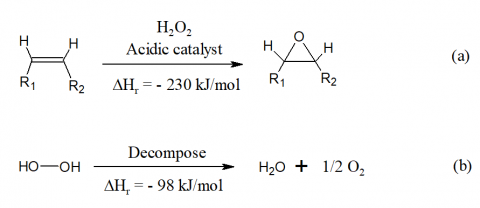Figure 1. Two exothermic reactions in vegetable oil epoxidation. Individual schemes are labeled as: (a) Transformation of unsaturated double bond in vegetable oil into epoxy group, (b) Thermal decomposition of hydrogen peroxide into water and gaseous oxygen

Countercurrent plate heat exchanger (Figure 2) is commonly employed throughout oleochemical manufacturing facilities as it is capable of handling highly viscous fluids, has high heat transfer coefficient due to corrugation-enhanced turbulences [8, 9], and has low fouling. It is known that surface area, A required for a specific heat duty, Q is given as:

$A=\int_{0}^{Q} \frac{d Q}{U_{0} \Delta T}$(1)

where U0 is the overall heat transfer coefficient and ΔT is the difference between the hot and cold streams at a given point. Driving force in heat exchanger can be described as logarithmic-mean temperature difference (LMTD), Tlm, and in countercurrent configuration, LMTD is determined as:

$T_{l m}=\frac{\left(t_{1}^{\prime}-t_{2}^{\prime \prime}\right)-\left(t_{2}^{\prime}-t_{1}^{\prime \prime}\right)}{\ln \left(\frac{t_{1}^{\prime}-t_{2}^{\prime \prime}}{t_{2}^{\prime}-t_{1}^{\prime \prime}}\right)}$(2)

where (t1' - t2") is the temperature difference at one end of the exchanger and (t2' - t1") is the temperature difference at the other end. The U0 is largely dependent on the type of fluids where heat exchange occurred. LMTD calculation is convenient in practice as it is based on terminal temperature and assumes that heat exchanger operating at steady state and overall heat transfer coefficient is constant.

Rapid evaluation for heat transfer in epoxidation process become necessary in debottlenecking heat exchanger system. Identifying faulty heat exchanger in the heat exchanger network was a challenge when epoxidation plant is operational or in the absence of working built-in temperature monitoring device. Fast screening allows engineers to isolate malfunctioned heat exchanger and redistributed heat duty accordingly without plant downtime or turnaround. As infrared thermometer gained more accurate in recent years at competitive price, it is of interest to evaluate such method capability to deliver rapid evaluation on the suspected heat exchanger. Infrared method appears to be advantageous as being non-intrusive, fast response time, and has high sensitivity. Infrared thermometer is easy to handle and relatively cheaper.

## 2.pngFigure 2. Typical flow of fluids in single-pass countercurrent plate heat exchanger

2. Experimental Section

2.1 Materials

A digital infrared thermometer was provided by Rolson® (model 27279), and had a built-in laser pointer for exact location measurements, temperature range between 253 to 473 K, and accuracy of  ±2.5 %. The measurement required focal ratio (distance-to-spot, D:S) 5:1 area and recorded at emissivity, ε = 0.95.

Three single-pass (1-1) countercurrent plate heat exchangers were obtained from an established commercial plate heat exchanger manufacturer. The first and small plate heat exchanger was PHX-S (0.18 m2 total surface area, 11 plates) and attached to the main reactor in Multipurpose Reactor Pilot Plant with volume capacity 150 L. The second and big plate heat exchanger was PHX-B (18 m2 total surface area, 77 plates) and attached to the main reactor in Polyol Pilot Plant with volume capacity 1 ton. The third plate heat exchanger and served as control was PHX-C (2.4 m2 total surface area, 17 plates) and attached to the Polyol Pilot Plant. PHX-B and PHX-S had identical mixture composition coming from an epoxidation reactor (i.e. monooleate, performic acid) in the hot stream and cooling water in the cold stream. PHX-C had heat transfer from water-to-water system.

2.2 Measurement

Temperature measurement was carried out during operational of the epoxidation process. A small piece of white masking tape was attached on the nozzles of heat exchanger and left for 30 minutes to equilibrate before measurement by infrared thermometer. Temperature measurement via infrared method [10, 11] typically rely on Wien's displacement law which states $\lambda_{\max } T=2.898 \times 10^{-3} \mathrm{m} . K$  where λmax represents wavelength at which maximal spectral emissive power of a perfect black body. As metallic or shinny surfaces give inaccurate reading based on Kirchhoff's law, measurement on a small piece of white masking tape was used instead in this study. Temperature variation were recorded three times and averaged. Flow rate for each stream was measured by triplicate bucket method and averaged. The same procedure was repeated for each heat exchanger evaluation.

3. Results and Discussion

3.1 Critical heat exchanger parameters (LMTD, U0, and Fouling)

The challenge in controlling epoxidation process is narrow permissible temperature regime. In this study, the hot reactor effluent was only allowed to cool by 10 K and resulted in narrow LMTD (Table 1). Extending the temperature range would upset the reaction and impair the final quality of the epoxidized vegetable oil. Figure 3 describes the temperature-enthalpy (T-Q) diagram of the process and total heat exchanged was 375 kW. The slope from temperature-enthalpy diagram is the inverse of stream enthalpy flowrate, 1/(FCp), with Cmin was the hot stream. Minimum temperature approach (ΔTmin) was 21 K and occur on the cold side of heat exchanger. As shown on Table 1, LMTD (equation (2)) for PHX-B and PHX-S were comparable despite working at different heat duty.

Table 1. Evaluation on heat exchanger performance

 Heat Exchanger PHX-S PHX-B PHX-C System Vegetable oil derivative-to-water Vegetable oil derivative-to-water Water-to-water Condition Unfouled Fouled Fouled LMTD, Tlm (K) 8 8 15 Uo  (J/s.m2.K) 694 868 4275 NTU 0.40 1.29 2.20 Temperature ratio 0.76 0.80 0.63

## 3.png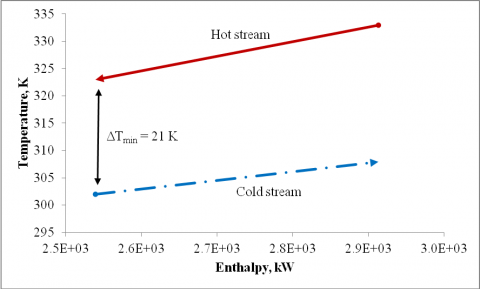Figure 3. Temperature-enthalpy (T-Q) diagram representing heat exchanger employed in epoxidation process

Table 2. The average possible error for the experimental parameters

 Equation for Uncertainty Uncer-tainty, % Re $\frac{\Delta R e}{R e}=\sqrt{\left(\frac{\Delta \dot{m}}{\dot{m}}\right)^{2}+\left(\frac{\Delta d_{e}}{d_{e}}\right)^{2}}$ 1.88 $T_{l m}$ $\frac{\Delta T_{l m}}{T_{l m}}=\sqrt{\left(\frac{\Delta t_{1}^{\prime}}{t_{1}^{\prime}}\right)^{2}+\left(\frac{\Delta t_{2}^{\prime \prime}}{t_{2}^{\prime \prime}}\right)^{2}+\left(\frac{\Delta t_{2}^{\prime}}{t_{2}^{\prime}}\right)^{2}+\left(\frac{\Delta t_{1}^{\prime \prime}}{t_{1}^{\prime \prime}}\right)^{2}}$ 1.56 U0 $\frac{\Delta U_{0}}{U_{0}}$$=\sqrt{\left(\frac{\Delta t_{1}^{\prime}}{t_{1}^{\prime}}\right)^{2}+\left(\frac{\Delta t_{2}^{\prime \prime}}{t_{2}^{\prime \prime}}\right)^{2}+\left(\frac{\Delta t_{2}^{\prime}}{t_{2}^{\prime}}\right)^{2}+\left(\frac{\Delta t_{1}^{\prime \prime}}{t_{1}^{\prime \prime}}\right)^{2}+\left(\frac{\Delta \dot{m}}{\dot{m}}\right)^{2}}$ 1.98 NTU $\frac{\Delta N T U}{N T U}=\sqrt{\left(\frac{\Delta t_{1}^{\prime}}{t_{1}^{\prime}}\right)^{2}+\left(\frac{\Delta t_{2}^{\prime}}{t_{2}^{\prime}}\right)^{2}+\left(\frac{\Delta t_{1}^{\prime \prime}}{t_{1}^{\prime \prime}}\right)^{2}+\left(\frac{\Delta \dot{m}}{\dot{m}}\right)^{2}}$ 1.69 Nu $\frac{\Delta N u}{N u}=\sqrt{\left(\frac{\Delta \dot{m}}{\dot{m}}\right)^{2}+\left(\frac{\Delta d_{e}}{d_{e}}\right)^{2}}$ 2.05

The values of U0 were experimentally determined using Q = U0A(Tlm) = Cmin(ΔT) relationship by utilizing specific heat capacity, Cp of reactor effluent. The temperature was obtained via the infrared thermometer. Note that for the analysis to be rigorous, the countercurrent heat exchanger would require number of channels per pass higher than 20. The detailed systematic error analysis was made based on the repeatability of the experiments and provided the accuracy of the experiments. The errors were based on the sensitivity of measuring devices and the least counts (Table 2). Values of uncertainty for Re was 1.88 %, LMTD was 1.56 %, U0 was 1.98 %, NTU was 1.69 %, and Nu was 2.05 %. This was in agreement with report demonstrated by Kandlikar  and Muthamizhi et al. . Improvement on the infrared measurement capability such as 2-dimensional infrared thermal imager would yield more accurate result and beyond the scope of this paper.

U0 for heat transfer from epoxidation effluent to cooling water in this process was determined to be 1600 J/s.m2.K without fouling and 868 J/s.m2.K for fouled plates. Heavy fouling was found primarily on the hot side of heat exchanger PHX-B with overall heat transfer resistance of 0.00039 m2.K/W. PHX-B had been in operational state for more than 7 years since previous major service. It was observed that PHX-S experienced reduction in stream flow rate by half on the hot side. Typically a slight pressure drop across plate heat exchanger was expected due to development of enhanced-corrugation turbulence with maximum allowable pressure drop at 0.2 bar. The mean channel pressure drop, ΔPchm can be determined as:

$\Delta P_{c h m}=\Delta P_{p o r t}-\Delta P_{e c}-\Delta P_{f, p i p e}$(3)

where ΔPport is the pressure drop between inlet and outlet ports in plate heat exchanger, ΔPec is related to expansion and contraction in enhanced-corrugation, and ΔPf,pipe is pressure drop due to friction in pipe connection. However, sudden change in cross-sectional area between process streams to channel port had increased flow maldistribution and affect hydraulic performance. Furthermore there were only 5 channels for the hot stream and 6 channels for the cold stream in PHX-S as compared to 38 channels for the hot streams and 39 channels for cold streams in PHX-B. It was known that plate heat exchanger experience momentous pressure drop for the first ten channels. However this detrimental effect is diminished by increasing number of channels . As PHX-S had number of channels less than 10 and relatively small ports diameter i.e. 20 mm as compared to 100 mm in PHX-B, the maldistribution effect was substantial and excessive pressure drop across the PHX-S was inevitable. Combined with end-plate effect , this had severely affected heat conduction [14, 15] and contributed lowered value of U0 than it was designed for. PHX-S was a brand new plate heat exchanger and unfouled, thus it was expected to have U0 approximately at 1600 J/s.m2.K instead of 694 J/s.m2.K (Table 1).

To further understand the heat transfer differences between PHX-B and PHX-S, it was of interest to determine the degree of convective heat transfer as prescribed in Nusselt number, Nu. For fully developed turbulent flow in plate heat exchanger and by applying Martin constants, Nu was determined as :

$N u=0.122 \operatorname{Pr}^{\frac{1}{3}}\left(\frac{\mu_{m}}{\mu_{w}}\right)^{\frac{1}{6}}\left(f \cdot R e^{2} \sin 2 \beta\right)^{0.374}$(4)

where Nu is Nusselt number, Pr is Prandtl number, μm is fluid viscosity at bulk fluid temperature, μw is fluid viscosity at heat transfer boundary surface temperature, f is friction factor for turbulence regime as defined from Focke et al.  and Bobbili et al. , Re is Reynolds number, and β is chevron angle. Heat transfer dimensionless parameters are tabulated on Table 3. PHX-S, PHX-B, and PHX-C had fully developed turbulent as all streams had values of Re larger than 400 [17, 18]. However values of Re for PHX-S were much smaller than PHX-B and might explained why it was underperformed. As Nu is a strong function of Re, PHX-S was found to have lower value of Nu and implied that it had lower degree of convection than PHX-B. PHX-B and PHX-C had larger values of Nu as both heat exchangers had very high values of Re. On the other hand, it was found that values of Pr (Pr = μCp/k) for PHX-B and PHX-S were comparable as it is known to be independent of geometry and velocity boundary in both heat exchangers were fully developed than thermal boundary.

Table 3. Heat transfer dimensionless parameters for plate heat exchanger employed in epoxidation process

 Heat Exchanger PHX-S PHX-B PHX-C System Epoxidized vegetable oil-to-water Epoxidized vegetable oil-to-water Water-to-water Reynolds,Re Hot side 1043 2197 5834 Cold side 1344 3053 4131 Prandtl,Pr Hot side 11.46 8.59 2.62 Cold side 7.97 4.97 2.61 Nusselt,Nu Hot side 32.68 49.77 65.51 Cold side 34.28 51.74 51.49

Fouling on the hot side of heat exchanger was responsible for the total heat transfer resistance, RTotal. RTotal is known to be inversely proportional with U0 ( $\left(R_{\text {Total}} \propto \frac{1}{U_{0} A}\right.$  ). Furthermore it was known that heat transfer resistance, Rtotal constituted by summation of heat transfer resistance on both sides, foulings resistance, and wall resistance:

$R_{\text {Total}}=R_{\text {hot}}+R_{f, h o t}+R_{\text {wall}}+R_{f, \text {cold}}+R_{\text {cold}}$(5)

where Rhot is the resistance on the hot side, Rf,hot is the fouling resistance on the hot side, Rwall is heat transfer resistance of the wall, Rf,cold is the fouling resistance on cold side, and Rcold is the resistance on the cold side. In order to determine degree of fouling, we employed Aspen EDR program under Design mode. Figure 4 denotes heat transfer resistance from the hot stream to the cold streams. Fouling on the hot side of heat exchanger constituted majority of the heat transfer resistance and accounted nearly half of overall heat transfer resistance. Deposits such as dirt, biological foul, and scales had formed on the heat exchange surface and introduced additional heat transfer resistance which reduced U0. Thus it needed more than twice surface area, A to accommodate such foulings. Values of  U0  for  PHX-B  were

determined to be as high as 1600 J/s.m2.K for unfouled situation and as low as 740 J/s.m2.K for dirty plates. Unlike PHX-S which suffered from excessive pressure drop, the low value of U0 in PHX-B signaled the fouling build-up across the plates. Similar trend of heat transfer resistance due to fouling was found on PHX-C for water-to-water heat transfer, which never has undergone major service for more than 10 years. For RTotal = 0.0013 m2.K/W, the U0 for PHX-C was determined to be approximately 6,200 J/s.m2.K and 3100 J/s.m2.K for clean and dirty plates, respectively.

## 4a.png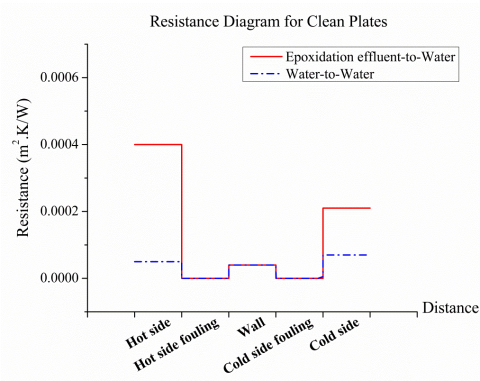## 4b.png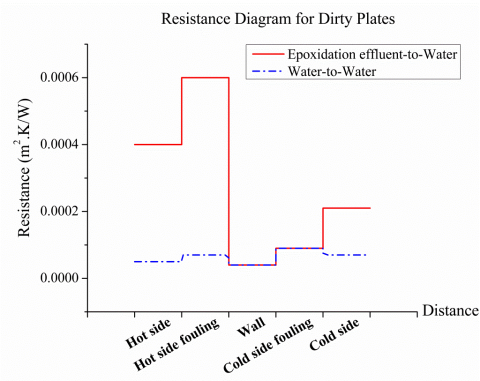Figure 4. Heat transfer resistance diagrams for PHX-B and and PHX-C

3.2 Number of heat transfer unit (NTU)

The effectiveness, E, of countercurrent heat exchanger was governed by number of heat transfer unit (NTU) and heat capacity ratio, Cr:

$E=\frac{1-\exp \left[-N T U\left(1-C_{r}\right)\right]}{1-C_{r} \exp \left[-N T U\left(1-C_{r}\right)\right]}$(6)

As Cr was a fixed parameter for epoxidation, effectiveness often relies on the value of NTU alone. NTU is expressed as:

$N T U=\frac{U A}{C_{\min }}$(7)

where Cmin was the limiting or minimum heat capacity flowrate, which in this analysis was the hot stream. There are several factors affecting NTU such as the plate gap, chevron angle, β, the pass arrangements and the heat flux.

## 5.pngFigure 5. Heat flux, q”, normal to a plate with thickness L

Consider the steady, two-dimensional (Figure 5) convection flow where heat transfer occurred from high temperature channel to low temperature channel, heat is neither generated nor destroyed, temperature gradient in y-axis is negligible, and heat loss to surrounding is negligible. Under these assumptions, the continuity equation is:

$\frac{d u}{d x}+\frac{d v}{d y}=0$(8)

$u \frac{d T}{d x}+v \frac{d T}{d y}=\alpha \frac{d^{2} T}{d x^{2}}$(9)

where u and v are the velocity component along the x-, y-axes. Further α is the thermal diffusivity. The boundary conditions are:

$\frac{d T}{d x}=\frac{-q^{\prime \prime}}{k}$ at $x=0$(10)

$v \rightarrow 0, T \rightarrow T_{\text {bulk}}$ as $x \rightarrow \infty$(11)

where k is the thermal conductivity of the plate. At time t with for incompressible fluid:

$\frac{d T}{d t}=\alpha \nabla^{2} \mathrm{T}$(12)

where by the definition the Laplacian operator is:

$\nabla^{2}=\nabla \cdot \nabla=\frac{\mathrm{d}}{\mathrm{dx}^{2}}+\frac{\mathrm{d}}{\mathrm{dy}^{2}}$(13)

Hence equation (12) become:

$\frac{d T}{d t}=\alpha\left(\frac{d^{2} T}{d x^{2}}+\frac{d^{2} T}{d y^{2}}\right)=\frac{k}{\rho c}\left(\frac{d^{2} T}{d x^{2}}+\frac{d^{2} T}{d y^{2}}\right)$(14)

where ρ is fluid density and c is specific heat.

The physical rate of heat transfer per unit area or heat flux, q” across a plate in Figure 5 was proportional to temperature gradient, ∇T across the plate:

$q^{\prime \prime}=-k \nabla \mathrm{T}=-\mathrm{k}\left[\frac{\mathrm{dT}}{\mathrm{dx}}+\frac{\mathrm{dT}}{\mathrm{dy}}\right]$(15)

Under steady state operation where temperature gradient existed in x-axis direction only and the dT/dy became negligible, equation (15) can be simplified to:

$q^{\prime \prime}=-\mathrm{k}\left[\frac{\mathrm{dT}}{\mathrm{dx}}\right]=-\mathrm{k}\left[\frac{\mathrm{T}_{\mathrm{low}}-\mathrm{T}_{\mathrm{high}}}{\mathrm{L}}\right]$(16)

Therefore the total heat transferred, Q, in a plate heat exchanger was dependent on the q”:

$q^{\prime \prime}=\frac{Q}{A}$(17)

The interaction between NTU (equation (7)) and q" (equation (17)) was unknown for oleochemical sector. Under some circumstances, design was limited by knowledge of NTU alone. NTU of 1.0 and less was regarded as low NTU and typically suitable for non-demanding process. On the other hand, NTU of 2.5 and above is considered as high NTU and often avoided as it is susceptible to excessive pressure drop. However we found that NTU = 1.29 met our requirements to effectively regulate the process at 333 K. NTU of 0.4 was severely suffered from process runaway. PHX-B (NTU = 1.29) was able to cool epoxidation reactor faster and more efficient in preventing reaction runaway than PHX-S (NTU=0.40). Shorter cooling duration was preferred in duration-sensitive process such as epoxidation. Lower NTU reflected lower value of q" that undermined temperature-sensitive epoxidation process. As duration for reactor cool down was a key parameter for heat exchanger employed in epoxidation process, it was further proposed that the heat exchanger performance for temperature-sensitive process to be better described as q".

To further understand this concept, we employed Aspen EDR to simulate the plate heat exchanger under Design mode and 1-1 pass arrangement. A total of 9 heat exchangers with total surface area between 18 m2 and 48 m2 and NTU between 0.36 and 1.67 were evaluated. Based on the EDR and experimental results, we compared the values of q” and Nu (Figure 6 and Figure 7, respectively). It is interesting to note that NTU had a sigmoid relationship with the q" for the countercurrent plate heat exchanger (Figure 6). Nonlinear regressions based on sigmoidally-shaped function were carried out in both figures using Gauss-Newton method (Table 4). The precision of analysis for both regressions were denoted as standard of error of regression, S at 1003 W/m2 and 1.05, respectively. Results from EDR were in harmony with experimental data. The proportional relationship between q” and NTU also resemble the effectiveness-NTU relationship interrelated report . Note that this analysis was validated only for 1-1 pass arrangement with bounded 0.36 < NTU < 1.67.

## 6.png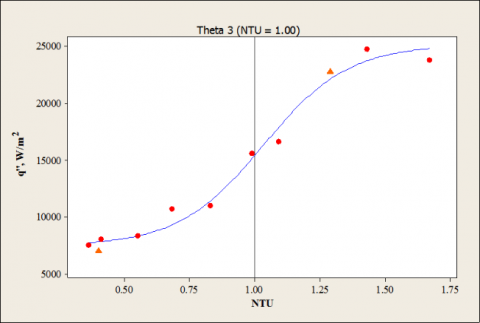Figure 6. NTU relationship with heat flux, q". Heat exchanger with higher NTU had higher transfer rate of heat per area, resulting in an intensive heat transfer. Individual legend is labeled as: Triangle = Experimental; Circle = EDR.

Table 4. Nonlinear regression parameters of function $\theta_{1}+\frac{\theta_{2}-\theta_{1}}{1+e^{\left(\frac{X-\theta_{3}}{\theta_{4}}\right)}}$ and 95 % confidence interval for Figure 6 and 7

 q" = q"(NTU) Nu = Nu (NTU) θ Estimate 95 % Confidence Interval Estimate 95 % Confidence Interval Lower Upper Lower Upper 1 25,201 23,159 28,756 49.973 48.448 51.663 2 7,452 4,557 8954 33.256 31.471 34.617 3 1.00 0.9 1.1 0.8423 0.786 0.903 4 0.20 0.1 0.3 0.0976 0.043 0.161
It was observed there was a critical junction in each Figure 6 and Figure 7 where q” and Nu changed significantly. These critical junctions were determined as θ3 in Table 4. Values of critical junction for both Nu=Nu(NTU) and q”=q”(NTU) were very close to each other. The critical points for Nu=Nu(NTU) occurred at NTU = 0.84 while for q”=q”(NTU) was at NTU = 1.00. It was hypothesized that the critical point due to greater effect of enhanced-corrugation heat transfer for fully-developed turbulence. For NTU < 0.84, the values of Re was far less than 2000. Therefore it was vital for plate heat exchanger employed in epoxidation process to operate above this critical junction and achieved Nu > 50. In retro perspective, one could approximate the critical junction for epoxidation based on value of q” when value of Nu is not readily available.

Common approaches to enhance NTU are to make multiple pass arrangement such as 2-1 as suggested by Kandlikar et al. , and by increasing the number of plates preferably more than 15 per pass. For epoxidation process, it was found that plate heat exchanger with NTU above 1.2 could expedite cooling of reaction mixture as countercurrent heat exchanger inherit higher efficiency at increasing capacity ratio . PHX-S was intentionally designed at reduced surface area and incapable to meet the heat duty requirement within stipulated cooling duration specified between 3 to 5 minutes. Despite PHX-B exchanged more heat than PHX-S, it generated less amount of entropy as indicated by temperature ratio $\left(\frac{t_{1}^{\prime \prime}}{t_{1}^{\prime}}\right)$ in Table 1. An ideal heat exchanger delivers maximum amount of heat and generates minimum amount of entropy when operating at ideal conditions . On the other hand, PHX-C had lower temperature ratio as it was used for capacity ratio at unity.

## 7.png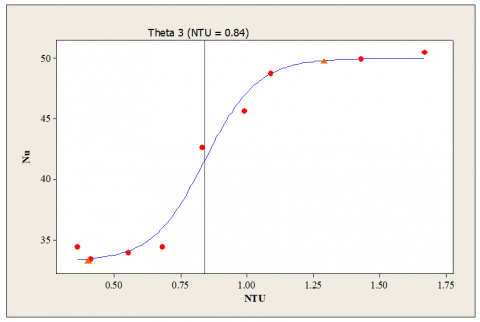Figure 7. Nusselt as a function of NTU. Individual legend is labeled as: Triangle = Experimental; Circle = EDR

4. Conclusions

Fast screening on plate heat exchangers performance in oleochemical application was accomplished by employing non-intrusive infrared thermometer. Indication of fouling was provided by comparing U0 with theoretical value (clean). To avoid excessive pressure drop which was not related to fluid friction, it was found that number of channels must be larger than 10. Performance of countercurrent plate heat exchanger was indicated by values of NTU and dimensionless Nu. NTU between 0.36 and 1.67 exhibited a nonlinear and sigmoidally-shaped curve relationship with heat exchanger performance needed for epoxidation process. It was demonstrated that plate heat exchanger with NTU > 1.25 and Nu > 50 was able to minimize runaway reaction in epoxidation.

Acknowledgment

The authors acknowledge Director-General of Malaysian Palm Oil Board (MPOB) for permission to publish the article. Special thanks to personnel at Process Engineering and Design Unit at MPOB for providing assistance in operating oleochemical pilot plants.

Nomenclature
 A surface area, m2 Cmin minimum heat capacity flowrate, J/(s.K) Cp stream heat capacity, J/(kg.K) Cr heat capacity ratio C specific heat de hydraulic diameter E effectiveness F stream flowrate, kg/hr f friction factor k thermal conductivity, J/(K.m.s) $\dot{m}$ mass flux, kg/(hr.m2) NTU number of heat transfer unit, dimensionless Nu Nusselt number, dimensionless Pr Prandtl number, dimensionless Q heat duty, W q” heat flux, W/m2 $R_{\text {Total}}$ total of heat transfer resistance, m2.K/W $R_{h o t}$ resistance on hot side, m2.K/W $R_{f, h o t}$ fouling resistance on hot side, m2.K/W $R_{\text {wall}}$ heat transfer resistance of wall, m2.K/W $R_{f, \text {cold}}$ fouling resistance on cold side, m2.K/W $R_{\text { cold }}$ resistance on cold side, m2.K/W Tlm logarithmic-mean temperature difference (LMTD), K $t_{1}^{\prime}$ inlet temperature of hot stream, K $t_{2}^{\prime}$ outlet temperature of hot stream, K $t_{2}^{\prime \prime}$ outlet temperature of cold stream, K $t_{1}^{\prime \prime}$ inlet temperature of cold stream, K $(\frac{t_{1}^{\prime \prime}} {t_{1}^{\prime}})$ temperature ratio, dimensionless U0 overall heat transfer, J/s.m2.K u, v velocity components along the x and y directions, respectively x, y Cartesian coordinates normal to and along the surface, respectively Greek symbols a thermal diffusivity, m2/s b chevron angle, ° ε emissivity ρ fluid density, kg/m3 θ regression parameter μm fluid viscosity at bulk fluid temperature, cP μw fluid viscosity at heat transfer boundary surface temperature, cP $\Delta H_{r}$ standard enthalpy of reaction, J/mol ΔPchm mean channel pressure drop in plate heat exchanger, bar ΔPport pressure drop between inlet and outlet ports in plate heat exchanger, bar ΔPec pressure drop due to expansion and contraction in enhanced-corrugation, bar ΔPf,pipe pressure drop due to friction in pipe connection, bar ΔT change in temperature stream, K ΔTmin minimum temperature approach, K $\nabla \mathrm{T}$ temperature gradient, K λmax wavelength at maximal spectral emissive power of a black body, μm
References

 Caltagirone, J. P., “Thermoconvective instabilities in a horizontal porous layer,” J. Fluid Mech., vol. 72, no. 2, pp. 269-287, 1975. DOI: 10.1017/S0022112075003345.

 T.W. Findley, D. Swern, J.T. Scanlan, “Epoxidation of unsaturated fatty materials with peracetic acid in glacial acetic acid solution,” Journal of the American Chemical Society, vol. 67, no. 3, pp. 412-414, 1945. DOI: 10.1021/ja01219a018.

 Z.S. Petrović, A. Zlatanić, C.C. Lava, S. Sinadinović-Fišer, “Epoxidation of soybean oil in toluene with peroxoacetic and peroxoformic acids — kinetics and side reactions,” European Journal of Lipid Science and Technology, vol. 104, no. 5, pp. 293-299, 2002. DOI: 10.1002/1438-9312(200205)104:5<293::aid-ejlt293>3.0.co;2-w.

 P. Lee, W. Yunus, W.M. Zin, S. Yeong, D.K. Abdullah, W. Lim, “Optimization of the epoxidation of methyl ester of palm fatty acid distillate,” Journal of Oil Palm Research, vol. 21, no. 2, pp. 675-682, 2009.

 A. Salmiah, R. Azman, I. Zahariah, “Epoxidized palm oil and palm stearin as plasticizers and stabilizer,” PORIM Report, PO 125(a) 87, 1987.

 E. Salzano, A.G. Agreda, V. Russo, M. Di., “Safety criteria for the epoxydation of soybean oil in fed-batch reactor,” Chemical Engineering, vol. 26, pp. 39-44, 2012. DOI: 10.3303/CET1226007.

 G.L. Matheson, O. Maass, “The properties of pure hydrogen peroxide-VI,” Journal of the American Chemical Society, vol. 51, no. 3, pp 674-687 , 1929. DOI: 10.1021/ja01378a004.

 G. Dieckelmann, H. Heinz, “Oxidation,” The Basics of Industrial Oleochemistry, Essen, Germany: Peter Pomp GmbH, 1988, pp. 133-142.

 Plate Heat Exchanger, by R. Blomgren, A. Knutsson, (2001), US Patent 6247528.

 Plate-Type Heat Exchanger,  by N. Tanaka, (2014), Patent WO2014155837.

 J.G. Christie, “Introduction to radiation heat transfer,” Transport Processes and Separation Process Principles, New Jersey, USA: Prentice Hall, 2003, pp. 301-307.

 G. Carlomagno, G. Cardone, “Infrared thermography for convective heat transfer measurements,” Experiments in Fluids, vol. 49, no. 6, pp. 1187-1218, 2010. DOI: 10.1007/s00348-010-0912-2.

 S. Kandlikar, R. Shah, “Multipass plate heat exchangers—effectiveness-NTU results and guidelines for selecting pass arrangements,” Journal of Heat Transfer, vol. 111, pp. 300-313, 1989. DOI: 10.1115/1.3250678.

 K. Muthamizhi, P. Kalaichelvi, “Development of Nusselt number correlation using dimensional analysis for plate heat exchanger with a carboxymethyl cellulose solution,” Heat and Mass Transfer, vol. 51, no. 6, pp. 815-823, 2015. DOI: 10.1007/s00231-014-1455-5.

 P.R. Bobbili, B. Sunden, S.K. Das, “An experimental investigation of the port flow maldistribution in small and large plate package heat exchangers,” Applied Thermal Engineering, vol. 26, pp. 1919-1926, 2006. DOI: 10.1016/j.applthermaleng.2006.01.015.

 N. Srihari, B. Prabhakara Rao, B. Sunden, S.K. Das, “Transient response of plate heat exchangers considering effect of flow maldistribution,” International Journal of Heat and Mass Transfer, vol. 48, no. 15, pp. 3231-3243, 2005. DOI: 10.1016/j.ijheatmasstransfer.2005.02.032.

 H. Martin, “A theoretical approach to predict the performance of chevron-type plate heat exchangers,” Chemical Engineering and Processing: Process Intensification, vol. 35, no. 4, pp. 301-310, 1996. DOI: 10.1016/0255-2701(95)04129-X.

 W. Focke, J. Zachariades, I. Olivier, “The effect of the corrugation inclination angle on the thermohydraulic performance of plate heat exchangers,” International Journal of Heat and Mass Transfer, vol. 28, no. 8, pp. 1469-1479, 1985. DOI: 10.1016/0017-9310(85)90249-2.

 F.-B. Liu, Y.-C. Tsai, “An experimental and numerical investigation of fluid flow in a cross-corrugated channel,” Heat and Mass Transfer, vol. 46, no. 5, pp. 585-593, 2010. DOI: 10.1007/s00231-010-0605-7.

 S.G. Kandlikar, R.K. Shah, “Asymptotic effectiveness-NTU formulas for multipass plate heat exchangers,” Journal of Heat Transfer, vol. 111, no. 2, pp. 314-321, 1989. DOI: 10.1115/1.3250679.

 A. Fakheri, “Heat exchanger efficiency,” Journal of Heat Transfer, vol. 129, no. 9, pp. 1268-1276, 2007. DOI: 10.1115/1.2739620.